f
Neutrium

# Welcome to Neutrium

Neutrium is a knowledge base of engineering topics, centred mainly around chemical engineering design challenges faced by engineers in their daily work. We created Neutrium to bridge the gap between theory and practice. Feel free to ask a question, leave feedback or take a look at one of our in-depth articles.

Index of Dimensionless Numbers

Dimensionless numbers play an important role in analysing fluid dynamics and heat and mass transfer problems. They provide a method by which complex phenomena can be characterised, often by way of a simple, single number comparison. This article provides a summary of dimensionless numbers and the formulae used to calculate them.

Dimensionless Numbers and Dimensional Analysis

Dimensionless numbers play an important role in analysing fluid dynamics and heat and mass transfer problems. They provide a method by which complex phenomena can be characterised, often by way of a simple, single number comparison. This article provides a brief overview of the derivation and use of dimensionless numbers.

Sherwood Number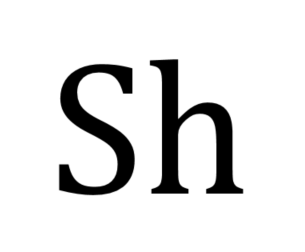The Sherwood number is a dimensionless number that represents the ratio of convective mass transfer to the rate of diffusive mass transport and is used in the analysis of mass transfer systems such as liquid-liquid extraction. This article describes the Sherwood number and typical formulations.

Schmidt Number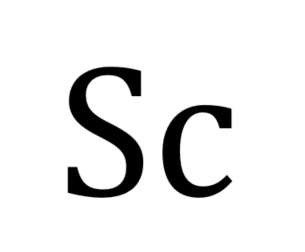The Schmidt number is a dimensionless number that describes the ratio of momentum diffusivity to mass diffusivity that is commonly used in analysis of mass transfer systems. This article describes the Schmidt Number and typical formulations.

Total Normal Emissivities of Selected Materials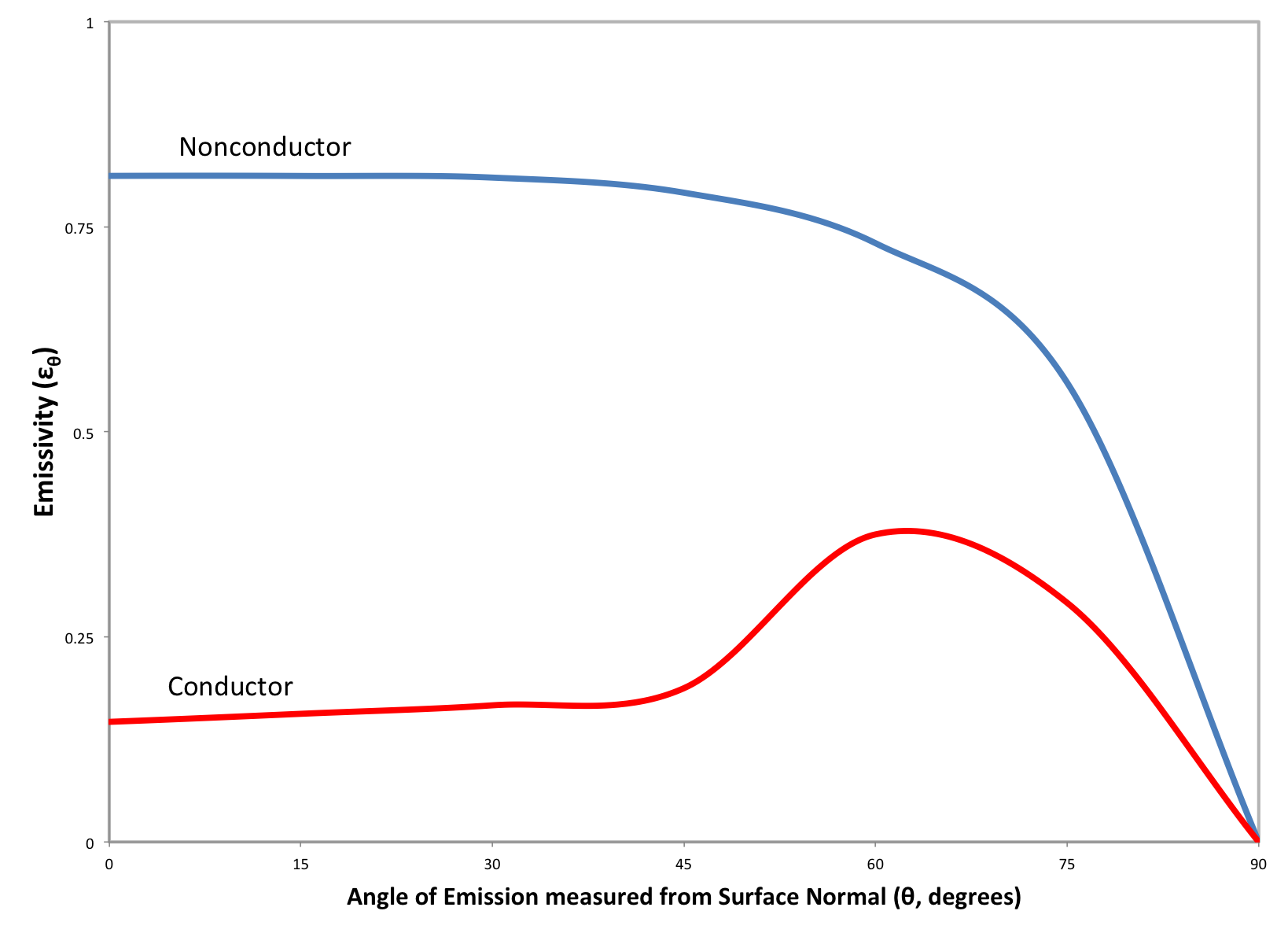Radiative heat transfer between two or more surfaces can be approximated using the total, normal emissivity. This article provides empirically determined total normal emissivities for a number of materials including metals, metal oxides, common building materials and paints.

Distillation Tray Efficiency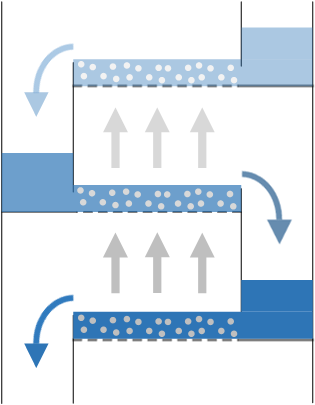Tray efficiency measures the performance of a distillation tray or trays against the maximum theoretical performance. Similarly, a concept called Height Equivalent to Theoretical Plate (HETP) is used to measure the performance in a packed column. This article describes methods of quantifying tray efficiency in distillation tray analysis.

Calculation of Emissivity for MetalsAt any given temperature, real materials emit less energy than that of a black body. The effectiveness of a material at emitting energy is represented by a radiative property called emissivity, which is the ratio of the actual energy emitted by the material to that of a black body at the same temperature. This article will provide an overview of the methods available for calculating the spectral, spectral-directional, hemispherical and total hemispherical emissivity for metals.

Relative Volatility and Activity Coefficients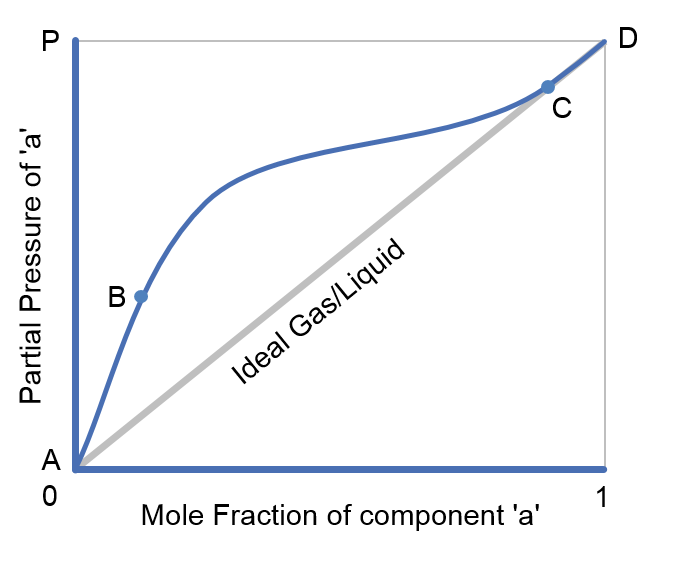Relative volatility is a comparative measure of the vapour pressures of components in a liquid mixture. It is commonly used in the design of absorption and separation processes such as distillation as it allows the difficulty of separating components to be quickly assessed.

Emissivity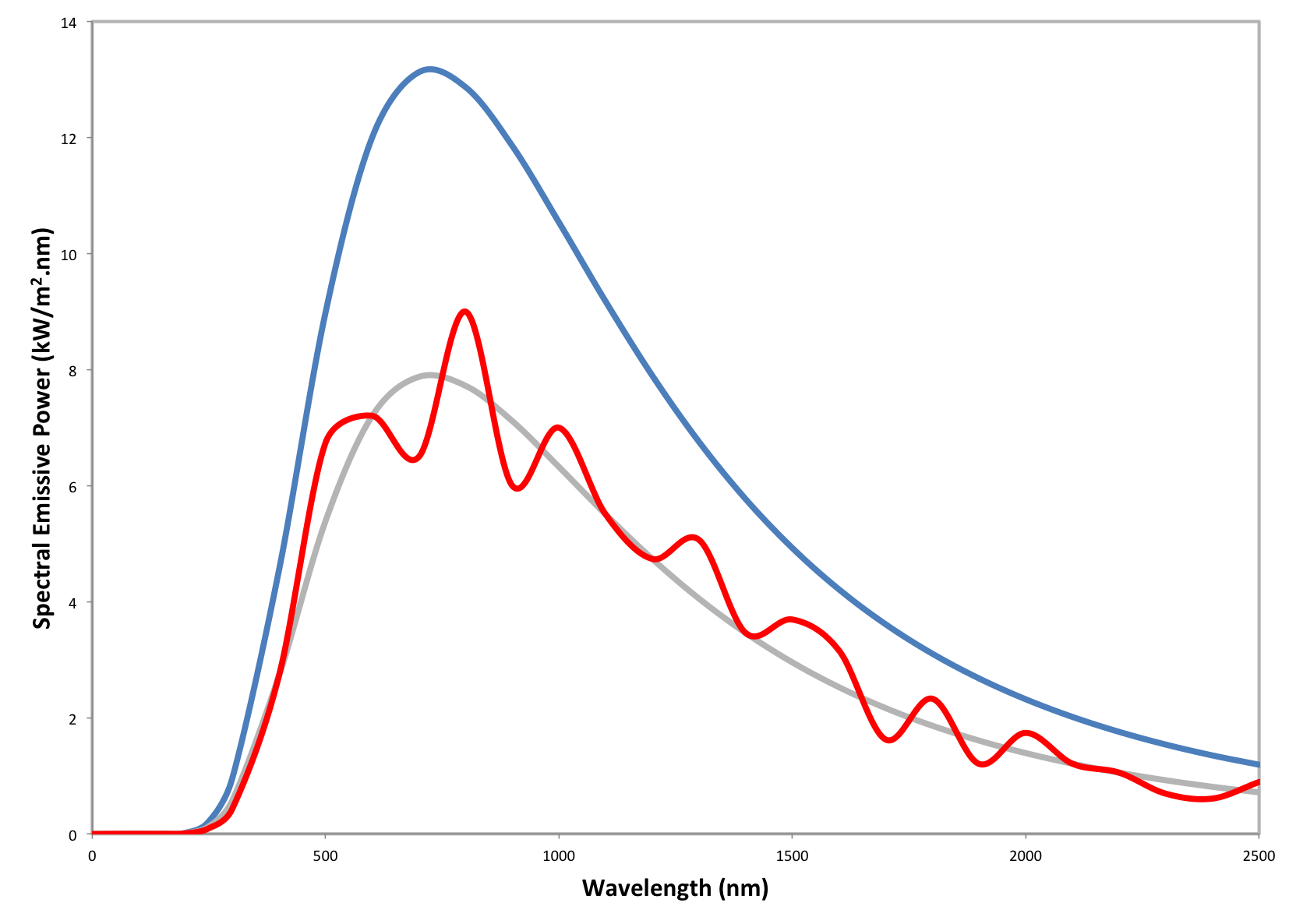At any given temperature, real materials emit less energy than that of a black body. The effectiveness of a material at emitting energy is represented by a radiative property called the emissivity factor, which is the ratio of the actual energy emission of the material to that of a blackbody at the same temperature. This article will provide an overview of emissivity and its many formulations.

McCabe-Thiele Plot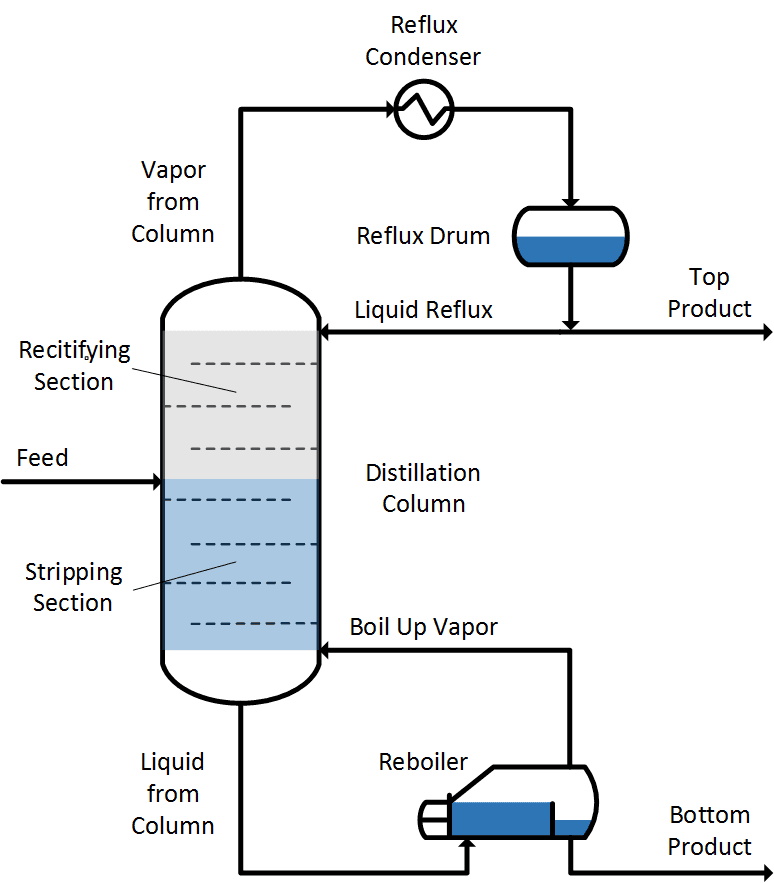A McCabe-Thiele plot is a simplified tool to assist in understanding distillation. It is a method for calculating the number of theoretical trays required for the distillation of a binary mixture. This article describes how to apply the McCabe-Thiele method.